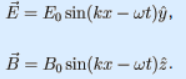# Problem: The simplest form of a traveling electromagnetic wave is a plane wave. For a wave traveling in the x direction whose electric field is in the y direction, the electric and magnetic fields are given by the picture shown above. This wave is linearly polarized in the y direction.1. In these formulas, it is useful to understand which variables are parameters that specify the nature of the wave. The variables Eo and Bo are the __________ of the electric and magnetic fields.a. maximab. wavelengthsc. amplitudes.d. velocities2. The variable ω is called the __________ of the wave.a. wavenumberb. wavelengthc. velocityd. frequency

###### FREE Expert Solution

1.

The maximum displacent of partcles by a wave is the amplitude.

96% (454 ratings)###### Problem DetailsThe simplest form of a traveling electromagnetic wave is a plane wave. For a wave traveling in the x direction whose electric field is in the y direction, the electric and magnetic fields are given by the picture shown above. This wave is linearly polarized in the y direction.

1. In these formulas, it is useful to understand which variables are parameters that specify the nature of the wave. The variables Eo and Bo are the __________ of the electric and magnetic fields.

a. maxima
b. wavelengths
c. amplitudes.
d. velocities

2. The variable ω is called the __________ of the wave.

a. wavenumber
b. wavelength
c. velocity
d. frequency